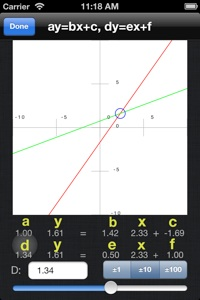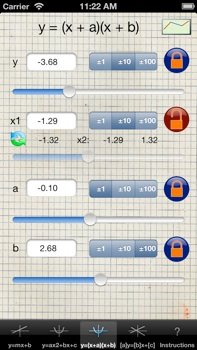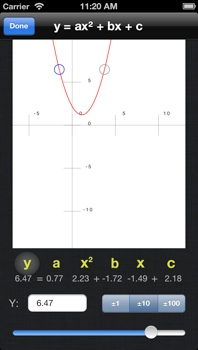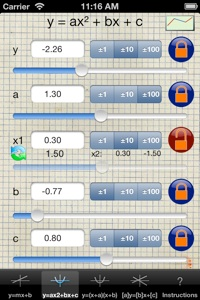Algebra HelperThis awesome calculator lets you compute and modify any of 4 different algebraic equations: linear (e.g. y=mx+b), quadratic polynomial (y=ax2+bx+c), quadratic factored (y=(x+a)(x+b)), and dual linear (y=mx+b,w=ux+v).

The convenient sliders let you see the effect of changing one of the independent parameters on the dependent one giving you a very natural understanding of how the relationship works.

In the plotting view the graph updates as you make changes to give an intuitive feeling and understanding of the equations.

Students, educators, researchers, and those interested in mathematics and business will all appreciate the convenience of seeing the computation recomputed at the touch of a slider.

AP Algebra Helper Demo Video

support and info: a2s2@mac.com

Radius Development LLC